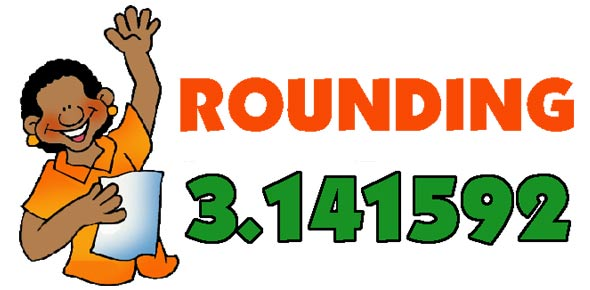Significant Digits And Rounding

15 Questions | Total Attempts: 17856SettingsHere is a quiz on using the rules for rounding and significant digits. For Mr. Wilson's class, please put your full name and class period in the box below. Your scores will be automatically recorded.

• 1.
Number of significant digits in 344.030
• A.

One

• B.

Four

• C.

Five

• D.

Six

• E.

Seven

• 2.
Number of significant digits in 55.40
• A.

One

• B.

Two

• C.

Three

• D.

Four

• E.

Five

• 3.
Number of significant digits in 0.0041500
• A.

One

• B.

Two

• C.

Three

• D.

Four

• E.

Five

• 4.
Number of significant digits in 2.04
• A.

One

• B.

Two

• C.

Three

• D.

Four

• E.

Five

• 5.
Number of significant digits in 0.08
• A.

One

• B.

Two

• C.

Three

• D.

Four

• E.

None

• 6.
Number of significant digits in 36
• A.

One

• B.

Two

• C.

Three

• D.

Four

• E.

Five

• 7.
Number of significant digits in 450 cm when the ruler is precise to 1 cm
• A.

One

• B.

Two

• C.

Three

• D.

Four

• E.

None

• 8.
Round off 10.1 to a whole number.
• A.

10

• B.

10.1

• C.

10.0

• D.

11

• E.

9

• 9.
Round off 119.357 to the tenths place.
• A.

119

• B.

120

• C.

118

• D.

119.3

• E.

119.4

• 10.
Round off 709 to the nearest ten's place.
• A.

709

• B.

710

• C.

711

• D.

709.1

• E.

3

• 11.
Round 7.009 to three significant digits.
• A.

7.00

• B.

7.009

• C.

7.01

• D.

7.10

• E.

7.11

• 12.
How many significant digits in 5.0000?
• A.

One

• B.

Two

• C.

Three

• D.

Four

• E.

Five

• 13.
How many significant digits in 0.0010?
• A.

One

• B.

Two

• C.

Three

• D.

Four

• E.

Five

• 14.
Round off 600.9 to three significant digits.
• A.

600

• B.

600.9

• C.

600.0

• D.

601

• E.

602

• 15.
Round off 7,877.0 to the nearest 100's place.
• A.

7,900

• B.

7,800

• C.

7,870

• D.

7,877

• E.

7,000

Related TopicsBack to top